# CFD waves project

FSID/SPH – STAR-CCM+ comparison of a 2D wave against a wall

In this article we will compare the impact forces and wave shape of a single wave clashing against a wall with data from two different papers. Both papers use different simulation methods for the liquid. One paper uses the Free surface Identification (FSID) numerical simulation code (non-linear 2D bi-fluid potential code developed by SLOSHEL JIP), the other paper uses the smooth particle hydrodynamics (SPH) method.

1. Simulations of breaking wave impacts on a rigid wall at two different scales with a two phase fluid compressible SPH model – P.M. Guilcher, L. Brosset, N. Couty, D. Le Touzé – 2013
2. Numerical study of density ratio influence on global wave shapes before impact – S. Etienne, Y-M. Scolan, L. Brosset – 2018

## Setup

In the image below the domain of the simulation is shown. Note that the wave shown in the image is for visualization only, and not the wave used. The domain is 2-dimensional with a height of 12m and a length of 20m. An elliptic ramp is added with a vertical half-dimension of 2.8m and a horizontal half-dimension of 18m.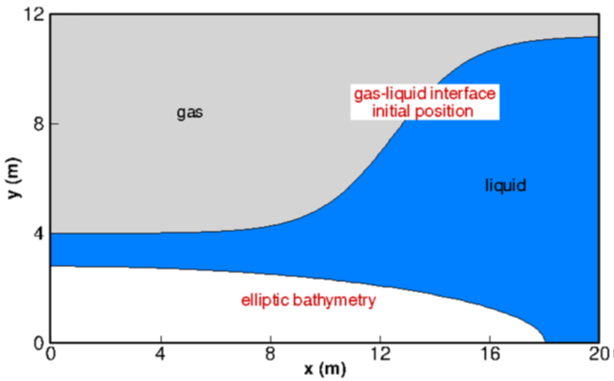Figure 1: wave domain [Etienne et. al. (2018)]

The wave is initialized in the domain using the equation: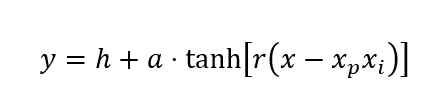With the initial values used in the example papers, this gives the following equation: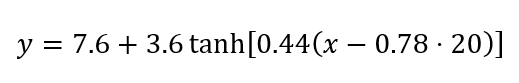The value of 0.78 for the xp variable is determined using the FSID results. Changing this value changes the waves, with larger or smaller gas bubbles (or no gas bubbles at all) due to the presence of the elliptic bathymetry. In this case, the value of 0.78 will give us a wave with a large gas bubble clashing on the wall.

Simcenter STAR-CCM+ 2020.1 was used for this simulation. The volume of fluid (VOF) method was used, with the air modelled as an ideal gas and water modelled as a constant density fluid, with a density of 1000 kg/m3. Gravity is present, with a value of 9.81 m/s2 in negative y-direction. The gravity, in combination with the ramp on the bottom, generates the wave.

## Mesh

For the mesh, a 2-dimensional polyhedral mesh is used. The cells near the impact wall (between x = 0m and x = 4m) have a size of 2.5cm while the cells in the rest of the domain have a size of 5cm.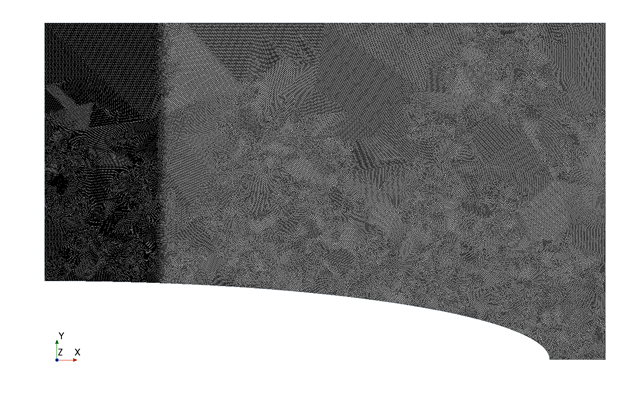## Results – pressure distribution

The area of interest for the wave impact is situated above a height of 4 meters and below 8 meters. Above 8 meter there is only air, without significant pressure changes. Between the 4- and 2.8-meter mark (2.8meter is the vertical half-dimension of the ramp) on the wall, water is present continuously. Because of this, no major pressure changes occur there in this simulation. Due to the above, these two areas are not evaluated.

In the two figures shown below, a comparison is made between our simulation performed in Simcenter STAR CCM+ 2020.1 and the simulation performed with SPH [Guilcher et al. (2013)]. The first pressure peak resulting from the STAR CCM+ simulation is located at the same location but results in a lower maximum pressure (4 bar versus 11 bar). However, decreasing the mesh size to 1cm in the area 0m < x < 4m increases the maximum pressure peak from Simcenter STAR CCM+ 2020.1 to 14 bar, indicating that the mesh size is important for the pressure peak.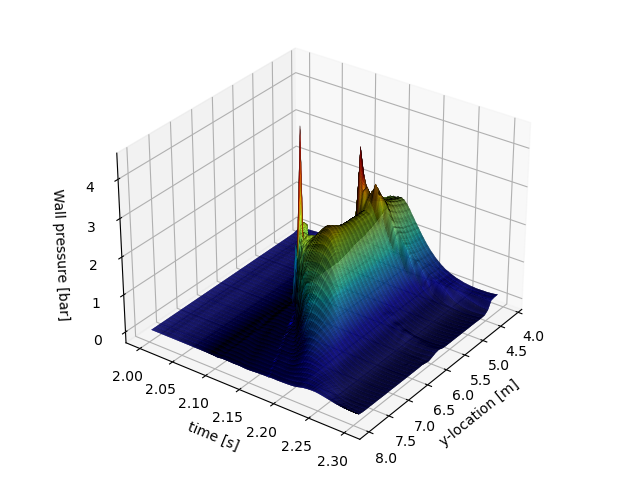Figure 2: Pressure distribution on the wall as generated with Simcenter STAR CCM+ 2020.1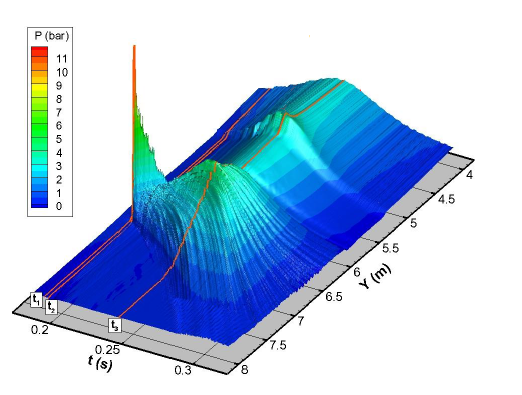Figure 3: Pressure distribution on the wall [Guilcher et. al (2018)]

In the pressure figure, three peaks can be identified.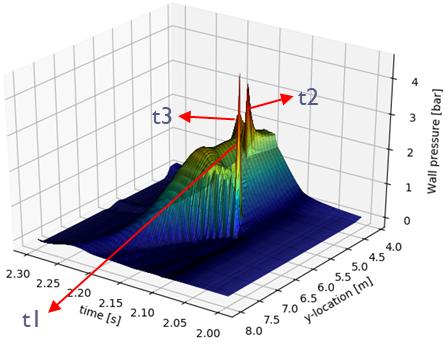1. The first peak is at 2.103s when the water first touches the wall at about 6m of height. This causes the highest pressure peak of 4.6 bar.
2. The second peak is at 2.138s, when the water coming from the wave crest pushes against the water coming from below, causing a slightly smaller high-pressure peak of 3.7bar.
3. At 2.155 the third peak can be identified, this peak is due to the interaction of the water and the gas bubble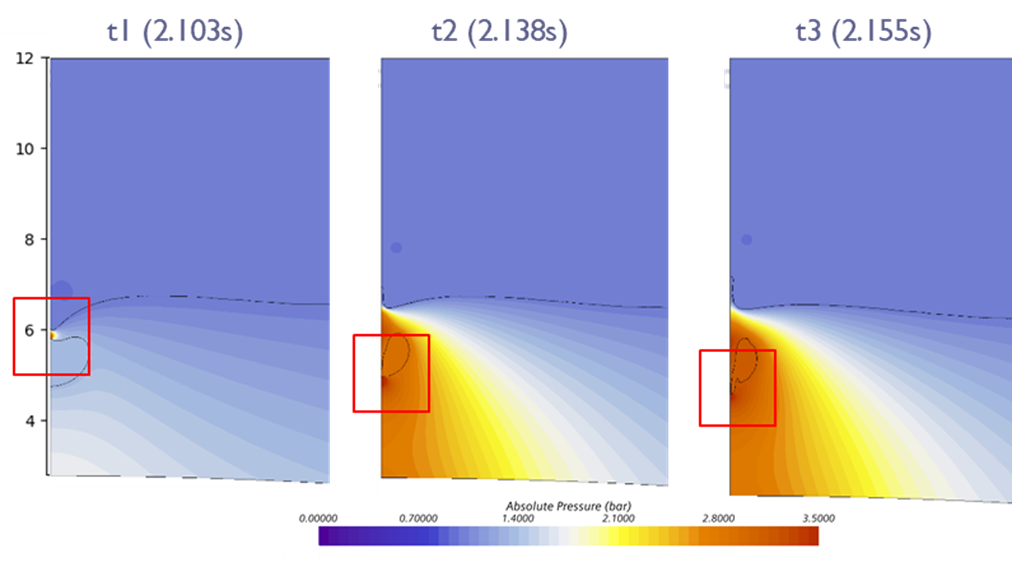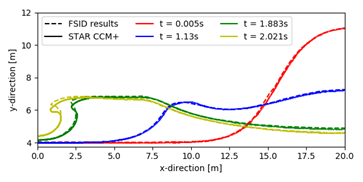## Results – Waveshape

In the images below the waveshape from the FSID simulation [Etienne et. al. (2013)] and Simcenter STAR CCM+ 2020.1 are given. The waveshapes are comparable, however in STAR CCM+ the wave crest moves down more than is the case in FSID.

## Conclusions

A close match in the wave shapes is found between FSID/SPH and STAR CCM+, with the wave generated by STAR CCM+ having a somewhat lower wave crest. The first impact pressure peak is somewhat lower as well, although this can also be due to the chosen mesh size.

March 23, 2021
get in touch